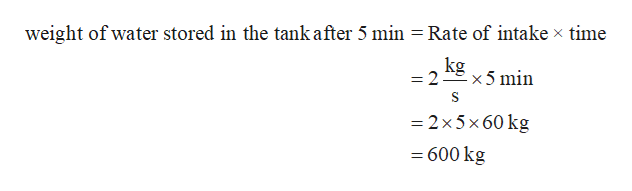# 2 kg/s2 kg/s1 kg/s(b)(а)

Question
124 views

Engineering Fundamentals – An Introduction to Engineering

6th Edition – Saeed Moaveni

Chapter 9 Length and Length- Related Variables in Engineering (Pages 286-311)

Question: How much water is stored after 5 minutes in each of the tanks on Example 9.5 (Page 303) if the volume of each tank is 14 cubic meters? Assume the density of water is 1000 kg/cubic meter.

Note the intake for both tanks is 2 kg/s

check_circle

Step 1

Given Information:

Intake of water in both of the tank = 2 kg/s

Volume of each tank = 14 m3

Density of water 1000 kg / m3

Step 2

Case 1: There is only intake of water in tank.

Step 3

Net weight of water stored in the tank...help_outlineImage Transcriptioncloseweight of water stored in the tank after 5 min Rate of intake x time kg -x 5 min =2 2x5x60 kg 600 kg fullscreen

### Want to see the full answer?

See Solution

#### Want to see this answer and more?

Solutions are written by subject experts who are available 24/7. Questions are typically answered within 1 hour.*

See Solution
*Response times may vary by subject and question.
Tagged in

### Civil Engineering